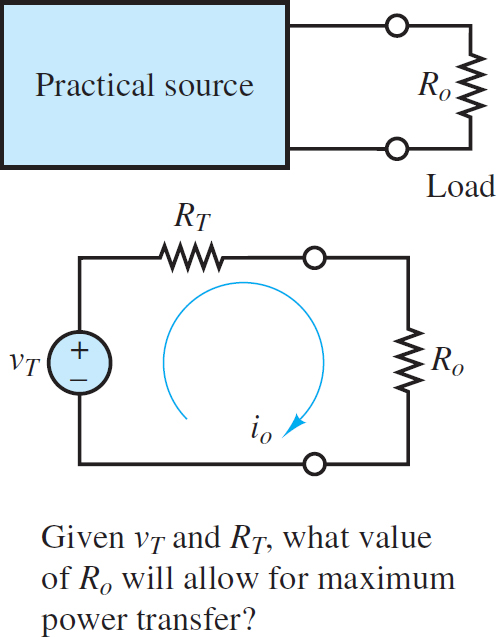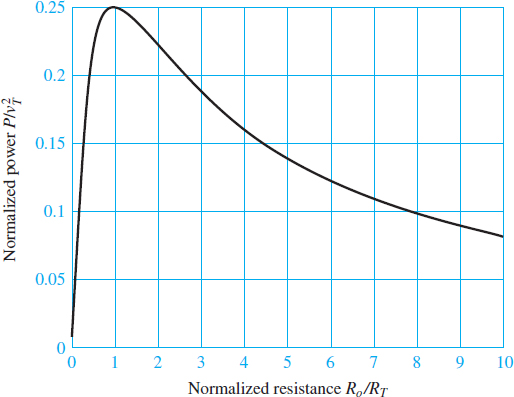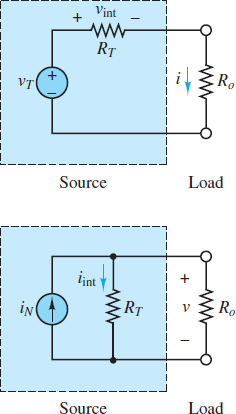Home / Electrical Circuits / Maximum Power Transfer Theorem

# Maximum Power Transfer Theorem

Want create site? Find Free WordPress Themes and plugins.

## Maximum Power Transfer Theorem Definition

Maximum power transfer theorem states that maximum power output is obtained when the load resistance Ro is equal to Thevenin resistance RT as seen from load Terminals.

The reduction of any linear resistive circuit to its Thevenin or Norton equivalent form is a very convenient conceptualization, as far as the computation of load-related quantities is concerned. One such computation is that of the power absorbed by the load.

The Thevenin and Norton models imply that some of the power generated by the source will necessarily be dissipated by the internal circuits within the source. Given this unavoidable power loss, a logical question to ask is, how much power can be transferred to the load from the source under the most ideal conditions? Or, alternatively, what is the value of the load resistance that will absorb maximum power from the source? The answer to these questions is contained in the maximum power transfer theorem, which is the subject of this section.Figure 1 Power transfer between source and load

## Maximum Power Transfer Theorem Derivation

The model employed in the discussion of power transfer is illustrated in Figure 1, where a practical source is represented by means of its Thevenin equivalent circuit. The power absorbed by the load Po is:

$\begin{matrix}{{P}_{o}}=i_{o}^{2}{{R}_{o}} & {} & \left( 1 \right) \\\end{matrix}$

$\begin{matrix}{{i}_{o}}=\frac{{{v}_{T}}}{{{R}_{o}}+{{R}_{T}}} & {} & \left( 2 \right) \\\end{matrix}$

Combining the two expressions, the load power can be computed as:

$\begin{matrix}{{P}_{o}}=\frac{v_{T}^{2}}{{{\left( {{R}_{o}}+{{R}_{T}} \right)}^{2}}}{{R}_{o}} & {} & (3) \\\end{matrix}$

The expression for Po can be differentiated with respect to Ro and set to zero to find the value of Ro that gives the maximum power absorbed by the load. (Here, VT and RT are assumed constant.)

$\begin{matrix}\frac{d{{P}_{o}}}{d{{R}_{o}}}=0 & {} & \left( 4 \right) \\\end{matrix}$

Plug in for Po and solve to obtain:

$\begin{matrix}\frac{d{{P}_{o}}}{d{{R}_{o}}}=\frac{v_{T}^{2}{{\left( {{R}_{o}}+{{R}_{T}} \right)}^{2}}-2v_{T}^{2}{{R}_{o}}\left( {{R}_{o}}+{{R}_{T}} \right)}{{{\left( {{R}_{0}}+{{R}_{T}} \right)}^{4}}} & {} & \left( 5 \right) \\\end{matrix}$

Thus, at the maximum value of Po the following expression must be satisfied.

$\begin{matrix}{{\left( {{R}_{o}}+{{R}_{T}} \right)}^{2}}-2{{R}_{o}}\left( {{R}_{o}}+{{R}_{T}} \right)=0 & {} & \left( 6 \right) \\\end{matrix}$

The solution of this equation is:

$\begin{matrix}{{R}_{o}}={{R}_{T}} & {} & \left( 7 \right) \\\end{matrix}$

So maximum power transferred is;

${{P}_{max}}=\frac{V_{T}^{2}}{4{{R}_{T}}}$

We got above expression by substituting RT=Ro into equation (3).

Thus, to transfer maximum power to a load, the equivalent source and load resistances must be matched, that is, equal to each other. Figure 2 depicts a plot of the load power divided by $v_{T}^{2}$ versus the ratio of Ro to RT. Note that this value is maximum when Ro = RT.Figure 2 Graphical representation of maximum power

This analysis shows that to transfer maximum power to a load, given a fixed equivalent source resistance, the load resistance must match the equivalent source resistance. What if the problem statement were reversed such that the maximum power transfer to the source resistance is sought for a fixed load resistance? What would be the value of the source resistance that maximizes the power transfer in this case?A problem related to power transfer is that of source loading. This phenomenon, which is illustrated in Figure 3, may be explained as follows: When a practical voltage source is connected to a load, the source current through the load will cause a voltage drop across the internal source resistance vint; as a consequence, the voltage actually seen by the load will be somewhat lower than the open-circuit voltage of the source. As stated earlier, the open-circuit voltage is the Thevenin voltage. The extent of the internal voltage drop within the source depends on the amount of current drawn by the load. With reference to Figure 3, this internal drop is equal to iRT, and therefore the load voltage will be

$\begin{matrix}{{v}_{o}}={{v}_{T}}-i{{R}_{T}} & {} & \left( 8 \right) \\\end{matrix}$

It should be apparent that it is desirable to have as small an internal resistance as possible in a practical voltage source.

In the case of a current source, the internal resistance will draw some current away from the load because of the presence of the internal source resistance; this current is denoted by iint in Figure 3. Thus, the load will receive only part of the short-circuit current (the Norton current) available from the source:

$\begin{matrix}{{i}_{o}}={{i}_{N}}-\frac{v}{{{R}_{T}}} & {} & \left( 9 \right) \\\end{matrix}$

It is therefore desirable to have a very large internal resistance in a practical current source.

## Maximum Power Transfer Solved ExampleFind Ro

Solution

Let’s find VT first across 150 Ω resistance

${{V}_{T}}=360*\frac{150}{150+30}$

${{V}_{T}}=300~V$

To find RT , short circuit the voltage source

${{R}_{T}}=150~||~30=25\Omega$

So, for maximum power transfer, we know that

${{R}_{o}}={{R}_{T}}=25\text{ }\Omega$

Now, Find Maximum power transfer to the load

${{P}_{max}}=\frac{V_{T}^{2}}{4{{R}_{T}}}=900~W$

Did you find apk for android? You can find new Free Android Games and apps.